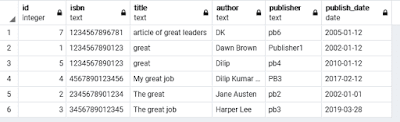### Introduction of datatypes in PostgreSQL

Before going to learn the next steps like create database, table, function and its other thing we have to know about the datatypes, so in this article we will learn about the datatypes. It will be beneficial for us when we are doing architecture design in PostgreSQL, it will give several benefits like consistency, Validation, Compactness, and performance. Consistency means we have to choose proper datatype for a column so that we can get the consistent and fastest result. Validation means, if we use proper datatype for a column we can easily validate data that is out of scope from the outside. Compactness means, we will use appropriate datatype as per required data, if data comes in single value then we have to choose data type accordingly. Proper data type according to data scope will increase the performance which is a very important factor in any database architecture design. PostgreSQL supports a wide set of the datatype, it also provides the facility to create user-defined datatype as per user requirement.
Let’s start a brief description of data types in PostgreSQL.
smallint: it is small range integer, can store 2bytes of data which range is -32768 to +32767.
integer: its storage size is 4bytes and range is -2147483648 to +2147483647
bigint: it is large range integer; the range is -9223372036854775808 to 9223372036854775807.
decimal: the range is up to 131072 digits before the decimal point; up to 16383 digits after the decimal point.
numeric: the range is up to 131072 digits before the decimal point; up to 16383 digits after the decimal point.
real: 4bytes storage size and range is 6 decimal digits precision.
double precision: 8-bytes storage size and range is 15 decimal digits precision.
smallserial: 2-byte storage size and range is 1 to 32767.
serial: 4 bytes storage size and the range is 1 to 2147483647.
bigserial: 8 bytes storage size and the range is 1 to 9223372036854775807.
money: 8 bytes storage size and the range is -92233720368547758.08 to +92233720368547758.07.
------Character type--------
character varying(n): Variable-length bit string, variable length with the limit.
character varchar(n): Variable-length bit string, variable length with the limit.
character(n): fixed-length character string.
char(n): fixed-length character string.
-------Binary Data Types---------
bytea: 1 or 4 bytes plus the actual binary string storage size
-------Date/Time data types---------
timestamp[(p)][without time zone]: 8 bytes storage size, it's contain both date and time(no time zone).
timestamp[(p)][with time zone]: 8 bytes storage size, it's contain both date and time(with  time zone).
date: 4 bytes storage size, its contains date only (not a time of the day)
time[(p)][without time zone]: 12 bytes storage size, it contains times of demurely without a time zone.
time[(p)][with time zone]: 12 bytes storage size, it contains times of demurely with the time zone.
interval [fields ] [(p)]: 12 bytes storage size it contains time intervals.
------Boolean Type------
boolean: 1-byte storage size, state of true or false.
-------- Geometric Type-------
geometric data types represent two-dimensional spatial objects
point: 16 bytes storage size, it contain the coordinates of the two-dimensional blocks. For example (x, y) x is latitude and y is longitude, x and y are floating points.
line: 32 bytes storage size, the line connects the two points, so this data types column contains the two points as like [(x1, y1), (x2, y2)].
lseg: 32 bytes storage size, the line connects the two points, so this data types column contains the two points as like [(x1, y1), (x2, y2)] online. Suppose (x1,y1) and (x2, y2) is line points and (x4, y4) and (x5,y5) belongs to the line, it means (x4, y4) and (x5, y5) is a segment of the line.
box: 32 bytes storage size, box means a geometrical draw of rectangular and square. boxes are represented by pairs of points that the opposite corner of the box value of type box is specified using the sentence like ((x1, y1), (x2, y2)).
Where (x1, y1) and (x2, y2) are two opposite corners of the box.
path: 16 bytes storage size and can be extended as 16+16n, path are represented by the list of connected. Fast can be open where the first and last points in the list are considered not committed or closed where the first and last points are considered connected.
[(X1, Y1), (X2, Y2),…….(Xn, Yn)]
polygon: 40 + 16n storage size, polygons are very similar to the closed path but are stored differently and have their own set of support routines
[(X1, Y1), (X2, Y2),…….(Xn, Yn)]
circle: 24 bytes storage size, Circle means, a point and a radius and used like as <(x1, y1), r>.